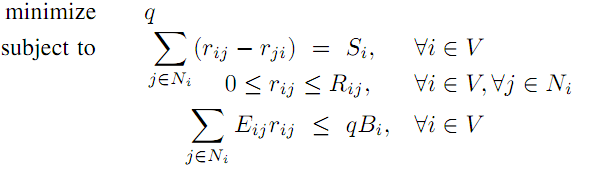# Formulate problem in CVX and using subgradient algorithm

I have this problem to solve in CVX, I would appreciate somebody to correct my error.

1.The life time of a node under flow r is defined as:

$$T_i®=\frac{B_i}{\sum_{j\in N_i}E_{ij}r_{ij}}$$

1. Now the network lifetime as :

$$T_{net}®=min_{i\in N}T_i®$$

3.The following problem wants to find a flow in network that maximize lifetime(which is max(min_{i\in N}T_i(r)))
$$Maximize \ \ T_{net}® \ subject \ to \ \ \sum_{j\in N_i}(r_{ij}-r_{ji}) =S_i, \ \ \forall i \in V \ 0\le r_{ij} \le R_{ij}, \ \ \forall i \in V , \forall j \in N_i$$

1. another form of above problem with additional constraint
$$Maximize \ \ T \ subject \ to \ \ \sum_{j\in N_i}(r_{ij}-r_{ji}) =S_i, \ \ \forall i \in V \ 0\le r_{ij} \le R_{ij}, \ \ \forall i \in V , \forall j \in N_i \ T\sum_{j\in N_i}E_{ij}r_{ij}\le B_i, \ \forall i \in V$$

$$q=\frac1T$$

The author says the following problem is a linear programming that he want to solve in a distributed manner, but I want solve it as is, to test and learn:my basic implementation to test for two node is:
(this is a network so B_i is a vector and r_ij is a matrice, the same is true for other variables)

 B_i=;%initial battery energy of node i
r_ij=; %flow rate from i to j
r_ji=; %flow rate from j to i
S_i=[0.5]; %the rate at which information is generated at node i
E_ij=[0.005]; %The energy consumption for sending a packet from node i to node j
R_ij=; %the maximum flow that a link from node i to node j can support

m=1;n=1;

cvx_begin
variables S_i(1) E_ij(m,n) r_ij(m,n) r_ji(m,n) R_ij(m,n) q;
minimize q
subject to
sum(r_ij-r_ji)==S_i(1);
sum(0.005 * r_ij)<=q*B_i;
-r_ij<=0;
r_ij<= R_ij;
cvx_end


By the way, at the end I want to implement section 4 of this article page 3 and 4,
I’m a beginner both in CVX and optimization so I really appreciate any suggestion that is helpful to implement this subgradeint algorithm(a pseudo code will be fine).
Thanks.

update: I changed some variables, now it works but is my formulation correct in CVX?

Why are you declaring E_ij to be a variable? It’s a constant, isn’t it?

In case every transmission has the same energy consumption yes, which is correct in here, but in my real problem E_{ij}=c_1+c_2d^4 , so I need to declare it as variable. Anyway, I change it to constant but still get the error.update: it works now but I cannot say if its correct.

You will not be able to treat E_ij as a variable. That makes the problem non-convex. FAQ: Why doesn’t CVX accept my problem? [READ THIS FIRST]

I have the same problem with R_ij. It looks like it should be a constant, but it can in theory be a concave expression. I’m also skeptical of r_ji. I don’t think that is intended to be a separate variable but a rearrangement of r_ij.

I say again what I said on Math StackExchange. It is clear to me that you are unfamiliar not just with CVX but with MATLAB itself. For instance, do you know exactly what sum() is doing—what it will do on matrices? We really can’t give people MATLAB tutorials here.

I really appreciate your answers @mcg, I will try to make it correct. About sum() you’re right, I just saw the usage in cvx example’s but didn’t notice that I have matrices here. So it doesn’t do what I want at all.